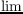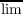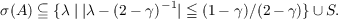#### Vol. 73, No. 1, 1977

 Download this articleFor screen For printingRecent Issues Vol. 325: 1  2 Vol. 324: 1  2 Vol. 323: 1  2 Vol. 322: 1  2 Vol. 321: 1  2 Vol. 320: 1  2 Vol. 319: 1  2 Vol. 318: 1  2Online Archive Volume: Issue:The Journal Subscriptions Editorial Board Officers Contacts Submission Guidelines Submission Form Policies for Authors ISSN: 1945-5844 (e-only) ISSN: 0030-8730 (print) Special Issues Author Index To Appear Other MSP Journals
Mercerian theorems via spectral theory

### Frank Peter Anthony Cass and Billy E. Rhoades

Vol. 73 (1977), No. 1, 63–71
##### Abstract

Given a regular matrix A, Mercerian theorems are concerned with determining the real or complex values of α for which αI + (1 α)A is equivalent to convergence. For α1, the problem is equivalent to determining the resolvent set for A, or, determining the spectrum σ(A) of A. where σ(A) = {λA λI is not invertible}. This paper treats the problem of determining the spectra of weighted mean methods; i.e., triangular matrices A = (ank) with an k = pk∕Pn, where p0 > 0, pn 0, k=0npk = Pn. It is shown that the spectrum of every weighted mean method is contained in the disc {λ|λ 12|12} (Theorem 1), and, if limpn∕Pn exists,

 σ(A) = {λ∣|λ − (2 − 𝜖)−1|≦ (1 − 𝜖)∕(2 − 𝜖)} ∪{pn∕Pn∣pn∕Pn < 𝜖∕(2 − 𝜖)},
where 𝜖 = limpn∕Pn.

Let γ =pn∕Pn, δ =pn∕Pn, S = {pn∕Pnn 0}. When γ < δ, some examples are provided to indicate the difficulty of determining the spectrum explicitly. It is shown that {λ|λ (2 δ)1|(1 δ∕(2 δ))}∪ S σ(A) andTheorem 1 is a generalization of the corresponding theorems of: S. Aljancic, L. N. Cakalov, K. Knopp, M. E. Landau, J. Mercer, Y. Okada, W. Sierpinski, and G. Sunouchi.

Primary: 40D25
##### Milestones